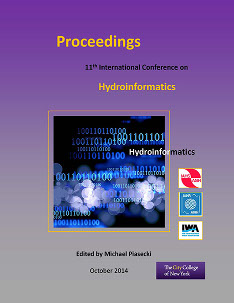## International Conference on Hydroinformatics

Presentation

8-1-2014

#### Abstract

For the design of a Water Distribution Systems (WDS) or for Residential Water Networks (RWN) different assumptions have to be made. This implies that the design methodologies for each of the two kinds of networks differ between them and so it is important to define a population value that limits the applicability of each method. This paper will present a new methodology for the RWN’s design process as well as the calculation the population limit. For designing the RWN demands’ predictions have to be made. The new methodology uses the concept of Poisson Rectangular Pulses (PRP) for modeling the water demand; this implies the use of the Binomial Poisson Distribution (BPD) which requires a significant amount of iterations and so, high computational cost. To reduce this, the methodology uses a simplification of BPD obtained by numerical methods. To find the population limit some networks were designed using a software call REDES (software developed by CIACUA) for many different demand flows which were equivalent to different population scenarios. This program uses OPUS methodology, a methodology for WDS’s design. Once the design process has ended, ideal diameter for each of the pipes where found, and it was necessary to generate an equation relation the population with the cost of the network. After the equation was established the design process was repeated but this time with RWN’s new methodology; for this purpose the software DisRed (software developed by CIACUA) was used. After this process important results were obtained. First of all a new software for RWN’s design process was developed, DisRed; this software’s results were optimal and obtained with low computational cost. In second place after doing the process previously described it was possible to calculate a limit population, this limit defines the range for which the new methodology has a valid application.